Home | English articles
From: Theor. Appl. Genet. 60, 97-98(1981)

A Remark on the Notions of Gene and Gametic Frequencies for Sex-linked Genes

G. Karigl

Department of Biomathematics, Technical University of Vienna, Vienna (Austria)

 Summary: A general model describing the evolution of genotypic frequencies at a heterosomal locus with n alleles is introduced. The treatment includes as a special case the standard derivation for X-linked loci as well as different concepts encountered in the literature which seem to contradict this derivation. The disagreement, however, is easily solved by distinguishing between gene änd gametic frequencies. Key words: Genetic model - Gene and gametic frequencies - Sex-linked genes

Introduction

The usual treatment of the inheritance of sex-linked genes in dioecious populations leads to oscillations in gene frequency rapidly approaching an equilibrium which yields the same value for both male and female individuals and depends on the initial frequency distribution. Moreover, at equilibrium, Hardy-Weinberg proportions prevail (in the female subpopulation). This behaviour has been well known since Jennings (1916) and Robbins (1918) who first considered numerical examples and the analytic solution, respectively, for two alleles.

Another approach was chosen by Richter (1979a, b, c) who derived different recursion relations resulting in two distinct limit values for the gene frequencies which he believes to be independent from the initial frequencies in males and females.

The discrepancy resulting from these different points of view can be resolved by considering a slightly generalized model for the inheritance of sex-linked genes which encompasses both conclusions mentioned above as simple corollaries. It turns out that all differences are caused by the use of the same terminology with a different meaning - as seems to be fairly often in situations of this kind and can be avoided by a differentiated use of the concepts of gene and gametic frequencies.

The General Model

Let us consider a X-linked heterosomal locus with alleles A1 , ..., An. Taking the males as the heterogamatic sex we denote the frequencies of Ai by xi in males and the frequencies of ordered types AiAj by Yij in females (i, j = 1 , ..., n). In doing so we assume that the first allele of type AiAj is always from the male parent. If we consider the whole population as a unit we have(1)

where xo =and Yo =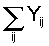denote the proportions of male and female individuals, respectively, in the population. We assume random mating and Mendelian segregation, i.e. the conditional probabilities for an offspring of typeor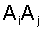given the mating type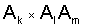of its parents can be written as(2a)

for males and(2b)

for females, whereif i = j and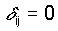otherwise. If there is no selection, the gene frequencies of the following generation are given by ( ‘ indicates frequencies in the daughter generation)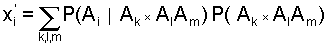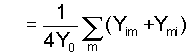(3a)

and similarly(3b)

An immediate consequence of Eqs. (3) iswhich implies the constant sex ratio of 1:1 in all subsequent generations. The analytic solution of the system of difference Eqs. (3) results in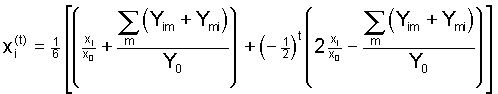,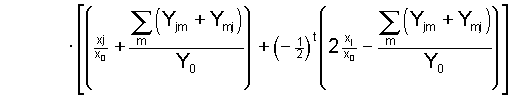,                (4)

whererefers to the generation number. This is the solution of our problem in terms of genotypic frequencies with respect to the whole population. The simplification to gene and gametic frequencies will be carried out in the following section. Concluding this paragraph we note that Hardy-Weinberg proportions hold forin the sense that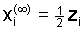and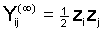, where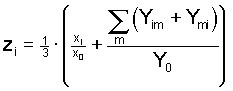.

Two Particular Cases

Let us now introduce gene frequencies pi and qj (of allele Ai) in the male and female subpopulation, respectively. Thenand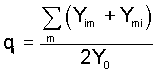such that. The recursions (3) imply the fundamental relations(5)

which can be encountered in different representations in the literature - at least for the two-allele case (Li 1976; Jacquard 1974; Nagylaki 1977). Furthermore, applying the solution (4) of the general system to this situation yields the well known outcome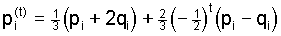,(6)

exhibiting damped oscillations around the common equilbrium value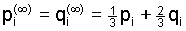which represents the overall gene frequency in the whole population.

A different point of view is obtained by considering gametic frequencies Pi and Qi (in males and females) defined as the proportions of gametes carrying the Ai allele with respect to all gametes (including those carrying the Y-chromosome), i.e.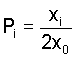andFollowing this idea we are led to the basic Eqs.(8)

Explicit formulas for the gametic frequencies can be derived in the same way as above and yield the limiting values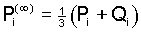,,                (9)

such that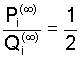Observing the discrepancy in Eqs. (5) and (8) and, consequently, in the limiting frequencies (7) and (9), it would seem to be useful to distinguish between the notions of gene and gametic frequencies, as both terms are common in two-locus theory. From the theoretical point of view both cases considered above are equivalent. Hence they do not represent contradictory theories but just different models describing the same phenomenon.

Literature

1. Jacquard, A. (1974): The Genetic Structure of Populations. Berlin, Heidelberg, New York: Springer
2. Jennings, H.S. (1916): The numerical result of diverse systems of breeding. Genetics 1, 53-89
3. Li, C.C. (1976): First Course in Population Genetics. Pacific Grove: Boxwood
4. Nagylaki, Th. (1977): Selection in one- and two-locus systems. Lecture Notes in Biomathematics, Vol. 15. Berlin, Heidelberg, New York: Springer
5. Richter, A. (1979a): Erbmäßig bevorzugte Vorfahrenlinien bei zweigeschlechtigen Lebewesen. Die Spaltungs-Proportionen in der Aszendenz bei geschlechtsgebundener Vererbung, erläutert am Beispiel des Menschen. Arch. Sippenforsch. 74, 96-109. Online: Deutsch | English
6. Richter, A. (1979b): Erbmäßig bevorzugte Nachfahrenlinien durch geschlechtsgebundene Vererbung. Die Spaltungs-Proportionen in der Deszendenz als Statistikwerte. (Online)
7. Richter, A. (1979c): There is not one common Hardy-Weinberg limiting value but two different ones for the relative X-chromosome gene frequencies in female and male individuals (unpublished)
8. Robbins, R.B. (1918): Some applications of mathematics to breeding problems II. Genetics 3, 73-92

 Dr. G. Karigl Department of Biomathematics Technical University of Vienna Argentinierstr. 8 A-1040 Vienna